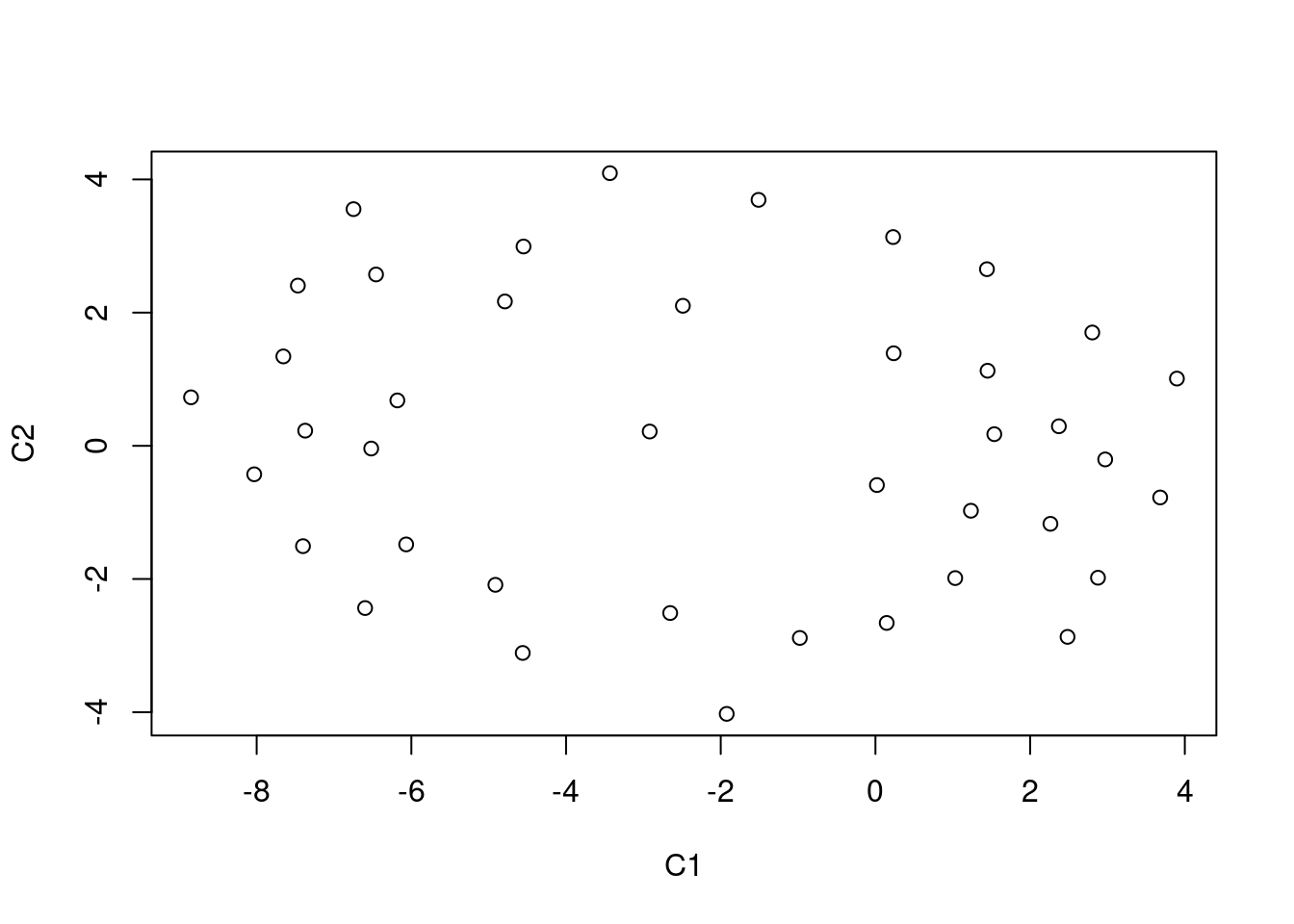# Installation

if(!requireNamespace("BiocManager", quietly = TRUE))
install.packages("BiocManager")
BiocManager::install("veloviz")

# Introduction

VeloViz creates RNA-velocity-informed 2D embeddings for single cell transcriptomics data. Inputs to VeloViz are the current and projected transcriptional states, which are obtained from RNA velocity analysis (e.g. using velocyto.R or scVelo).

We start by extracting the current and projected transcriptional states from the output of velocyto.R. An example velocity output is provided with VeloViz for illustration purposes.

data(vel)

curr <- vel$current #observed current transcriptional state proj <- vel$projected #predicted future transcriptional state

Now we can build the VeloViz graph using the current and projected transcriptional states using the VeloViz function buildVeloviz. User inputted parameters are explained at https://jef.works/veloviz/.

veloviz <-  veloviz::buildVeloviz(curr = curr, proj = proj,
normalize.depth = TRUE,
use.ods.genes = FALSE,
pca = TRUE, nPCs = 3,
center = TRUE, scale = TRUE,
k = 10, similarity.threshold = -1,
distance.weight = 1, distance.threshold = 1,
weighted = TRUE, verbose = FALSE)

From the VeloViz object, we can extract the 2D embedding coordinates for plotting.

emb.veloviz <- veloviz\$fdg_coords
plot(emb.veloviz)sessionInfo()